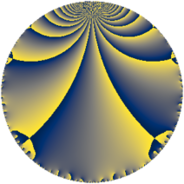# Properties

 Label 105.4.sLevel $105$ Weight $4$ Character orbit 105.s Rep. character $\chi_{105}(26,\cdot)$ Character field $\Q(\zeta_{6})$ Dimension $64$ Newform subspaces $2$ Sturm bound $64$ Trace bound $3$

# Learn more about

## Defining parameters

 Level: $$N$$ $$=$$ $$105 = 3 \cdot 5 \cdot 7$$ Weight: $$k$$ $$=$$ $$4$$ Character orbit: $$[\chi]$$ $$=$$ 105.s (of order $$6$$ and degree $$2$$) Character conductor: $$\operatorname{cond}(\chi)$$ $$=$$ $$21$$ Character field: $$\Q(\zeta_{6})$$ Newform subspaces: $$2$$ Sturm bound: $$64$$ Trace bound: $$3$$ Distinguishing $$T_p$$: $$2$$

## Dimensions

The following table gives the dimensions of various subspaces of $$M_{4}(105, [\chi])$$.

Total New Old
Modular forms 104 64 40
Cusp forms 88 64 24
Eisenstein series 16 0 16

## Trace form

 $$64q + 128q^{4} + 92q^{7} + 2q^{9} + O(q^{10})$$ $$64q + 128q^{4} + 92q^{7} + 2q^{9} + 330q^{12} + 40q^{15} - 752q^{16} - 182q^{18} - 396q^{19} - 474q^{21} + 408q^{22} - 144q^{24} - 800q^{25} + 700q^{28} + 140q^{30} + 1020q^{31} + 744q^{33} - 1224q^{36} - 1316q^{37} - 72q^{39} + 102q^{42} + 664q^{43} - 990q^{45} - 936q^{46} + 1960q^{49} + 1860q^{51} + 6756q^{52} + 6036q^{54} - 1632q^{57} - 2160q^{58} + 810q^{60} - 3324q^{61} - 1432q^{63} - 3904q^{64} - 5700q^{66} - 2596q^{67} - 900q^{70} + 4970q^{72} + 756q^{73} - 3792q^{78} - 652q^{79} - 1534q^{81} - 5832q^{82} - 9300q^{84} + 1440q^{85} - 6756q^{87} + 3336q^{88} + 4164q^{91} - 864q^{93} - 2304q^{94} + 11412q^{96} + 6256q^{99} + O(q^{100})$$

## Decomposition of $$S_{4}^{\mathrm{new}}(105, [\chi])$$ into newform subspaces

Label Dim. $$A$$ Field CM Traces $q$-expansion
$$a_2$$ $$a_3$$ $$a_5$$ $$a_7$$
105.4.s.a $$32$$ $$6.195$$ None $$0$$ $$-2$$ $$-80$$ $$46$$
105.4.s.b $$32$$ $$6.195$$ None $$0$$ $$2$$ $$80$$ $$46$$

## Decomposition of $$S_{4}^{\mathrm{old}}(105, [\chi])$$ into lower level spaces

$$S_{4}^{\mathrm{old}}(105, [\chi]) \cong$$ $$S_{4}^{\mathrm{new}}(21, [\chi])$$$$^{\oplus 2}$$